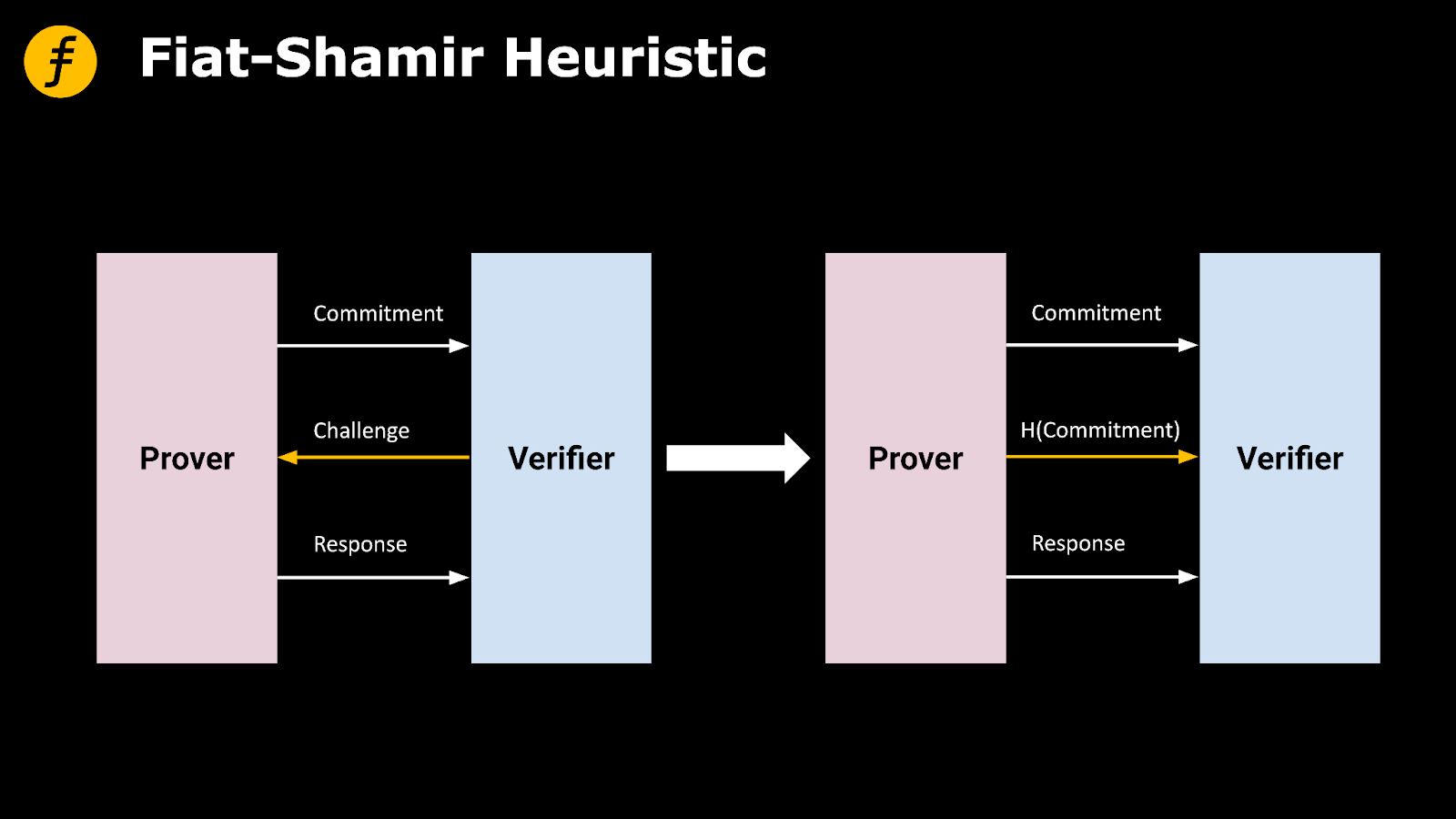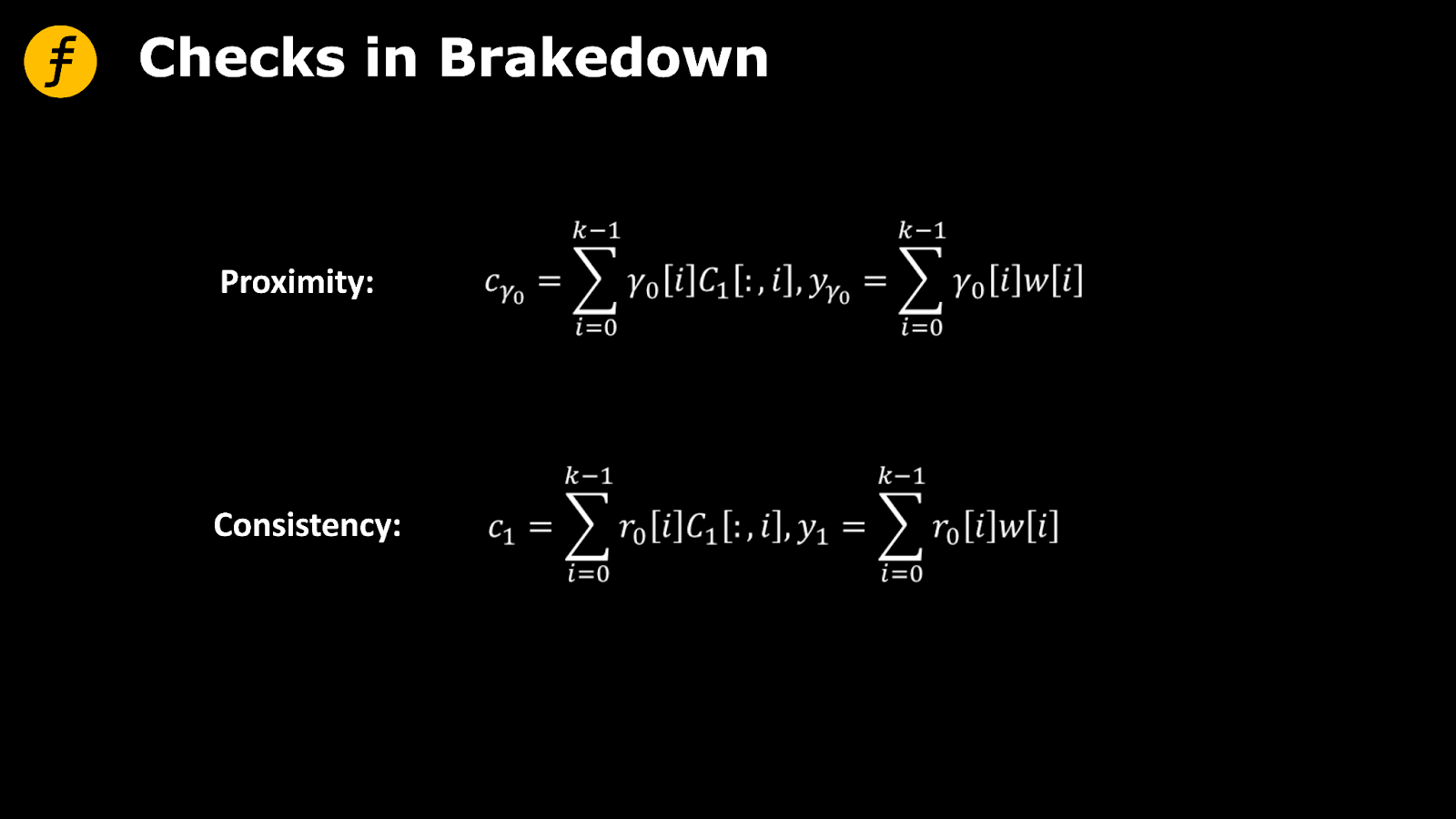/ 0评 / 0

# 哈希函数（Hash Function）

Fiat-Shamir 启发式（Heuristic）# 非交互式 FOAKS1. function PC. Commit(ϕ)：

2. Parse was a k × k matrix. The prover locally computes the tensor code encoding C1，C2 ，C1 is a k × n matrix，C2 is a n × n matrix.

3. for i ∈ [n] do

4. Compute the Merkle tree root Roott=Merkle.Commit(C2[:,i])

5. Compute a Merkle tree root R=Merkle.Commit([Root0,......Rootn-1]),and output R as the commitment.

6. function PC. Prover(ϕ, X, R)

7. The prover generates a random vector γ0 ∈ Fk by computing: γ0 =H(C1,R, r0,r1)

8. Proximity:9. Consistency:10. Prover sends c1,y1,cγ0,yγ0 to the verifier.

11. Prover computes a vector Î as challenge, in which Î = H(C1,R, r0,r1,c1,y1,cγ0,yγ0)

12. for idx ∈ Î do

13. Prover sends C1 [:,idx] and the Merkle tree proof of Rootidx for C2 [:,idx] under R to verifier

14. function PC. VERIFY_EVAL(ΠX,X,y= ϕ (X),R)

15. Proximity: ∀idx ∈ Î, Cγ0 [idx] == and Ec(yγ0) == Cγ0

16. Consistency: ∀idx ∈ Î, C1 [idx] == and Ec(y1) == C1

17. y==

18. ∀idx ∈ Î, Ec ( C1[:,idx]) is consistent with ROOTidx, and ROOTidx’s Merkle tree proof is valid.

19. Output accept if all conditions above holds. Otherwise output reject.

# 结语

## 参考文献

1.Fiat, Amos; Shamir, Adi (1987). "How To Prove Yourself: Practical Solutions to Identification and Signature Problems". Advances in Cryptology — CRYPTO' 86. Lecture Notes in Computer Science. Springer Berlin Heidelberg. 263: 186–194. doi:10.1007/3-540-47721-7_12. ISBN 978-3-540-18047-0.

2.https://www.cnblogs.com/zhuowangy2k/p/12246575.html

• 默认
• 护眼
• 夜晚
• Serif
• Sans